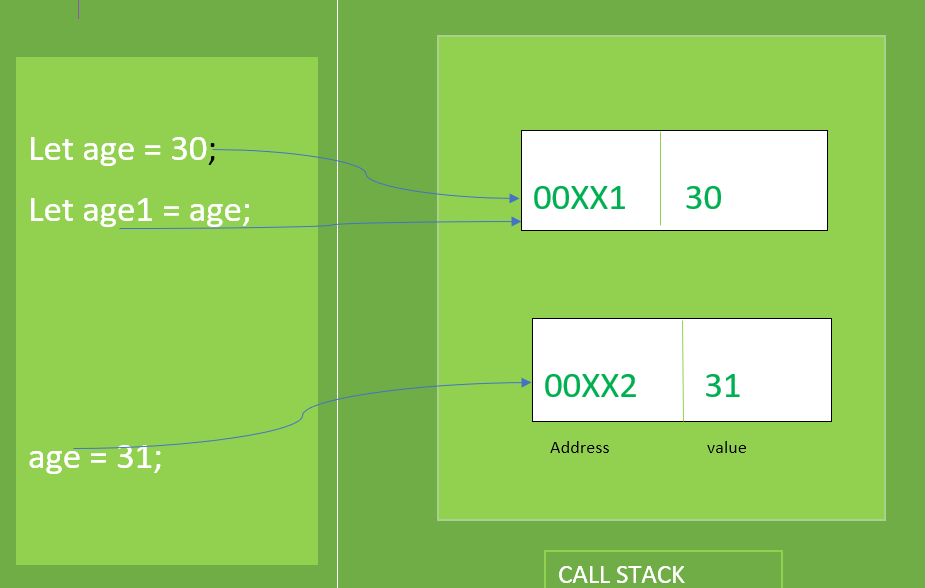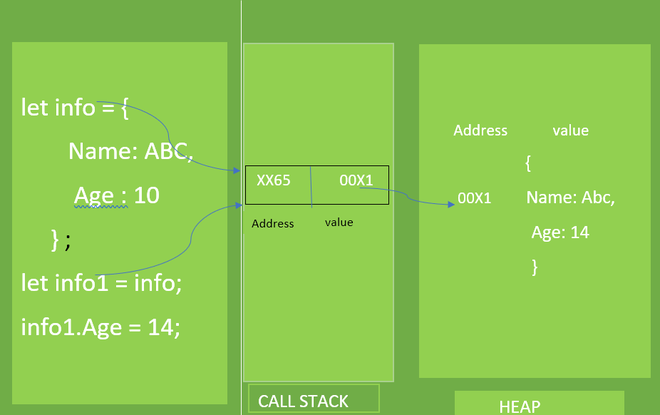GeeksforGeeks App
Open AppBrowser
Continue

# Primitive and Reference value in JavaScript

In JavaScript, a variable may store two types of values, Primitive values or Reference values. This article will describe and help to compare both these types of values.

Primitive value: JavaScript provides six types of primitive values that include Number, String, Boolean, Undefined, Symbol, and BigInt. The size of Primitive values are fixed, therefore JavaScript stores the primitive value in the call stack (Execution context).

When we access a primitive value, we manipulate the actual value stored in that variable. Thus, variables that are primitive are accessed by Value. When we assign a variable that stores a primitive value to another, the value stored in the variable is created and copied into the new variable.

Example: Let us take an example to understand primitive value:Primitive value

## Javascript

 `let age = 30;``let age1 = age; ``// Pointing to age`` ` `console.log(`age = \${age}  age1 = \${age1}`);`` ` `age = 31; ``// Pointing to new address`` ` `console.log(`age = \${age}  age1 = \${age1}`);`

Output:

``` age = 30  age1 = 30
age = 31  age1 = 30```

Reference Value: JavaScript provides three types of Reference values that include Array, Object, and Function. The size of a reference value is dynamic therefore It is stored on Heap.

When we access a reference value, we manipulate it through reference, not through its actual value that is stored. Thus, variables that are reference values are accessed by reference. When we assign a reference value from one variable to another, the value stored in the variable is also copied into the location of the new variable but the difference is that the values stored in both variables now are the address of the actual object stored on the heap. As a result, both variables are referencing the same object, So we can manipulate the original object from both variables.

Example: Let us take an example to understand reference value:Reference value

## Javascript

 `let info = {``    ``Name :``"Abc"``,``    ``Age :10``}``console.log(`Name : \${info.Name} Age : \${info.Age}`);`` ` `let info1 = info;``info1.Age = 14; ``// Change the Age of original object``console.log(`Name : \${info.Name} Age : \${info.Age}`);`

Output:

```Name : Abc Age : 10
Name : Abc Age : 14```

My Personal Notes arrow_drop_up
Related Tutorials